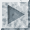[an error occurred while processing this directive]

## infinityinfinity /n./ 1. The largest value that can be represented in a particular type of variable (register, memory location, data type, whatever). 2. `minus infinity': The smallest such value, not necessarily or even usually the simple negation of plus infinity. In N-bit twos-complement arithmetic, infinity is 2^(N-1) - 1 but minus infinity is - (2^(N-1)), not -(2^(N-1) - 1). Note also that this is different from "time T equals minus infinity", which is closer to a mathematician's usage of infinity.